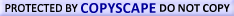# Cool math tricks

This unit is dedicated to showing you a variety of cool math tricks that will help you tackle some basic math problems with speed and great accuracy.

Multiplying by powers of ten
Learn to multiply by powers of ten the easy way with these useful shortcuts tricks

Multiplication by 11
Learn how to perform multiplication by 11 in 10 seconds or less with these useful tricks

Squaring numbers ending with five
What if you could square any number two digit number ending in five in 4 seconds or less?

Finger multiplication
Easy, straightforward, and quick multiplication with your fingers!

Number trick with 1089
impress your friends with this 1089 trick!

Compensation
Learn how to use compensation to quickly add, subtract, divide, and multiply

Fun math game: Become a mathionaire by answering math questions

Still struggling with fractions? Get rid of your fears and frustrations once and for all!

Buy my ebook. It offers a thorough coverage of fractions!WyzAnt Tutoring## Recent Articles1. ### solution word problem

Jan 08, 16 02:23 PM

A scientist wants to dilute a 60% acid solution by adding some 20% solution. If she starts with 80 ml of the 60% solution, how many milliliters of the